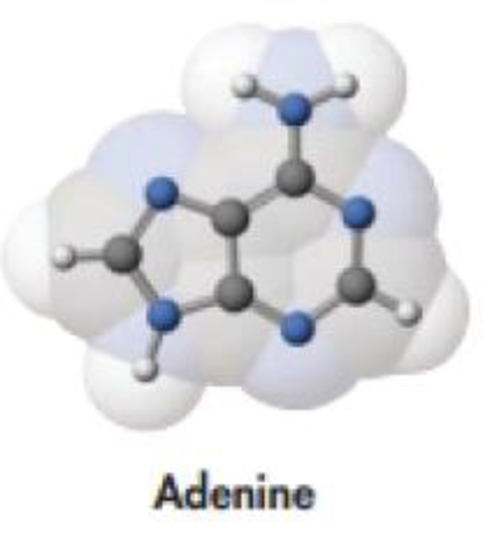Chapter 2, Problem 119GQ

Chapter
Section
Textbook Problem

The structure of one of the bases in DNA, adenine, is shown here. Which represents the greater mass: 40.0 g of adenine or 3.0 × 1023 molecules of the compound?Interpretation Introduction

Interpretation: It should be determined that which represents the greater mass, 40.0g of adenine or 3.0×1023 molecules of the compound.

Concept introduction:

• Amountofsubstance(mole)=GivenmassofthesubstanceMolarmass
• Massofthesubstance=Amountofthesubstance×Molarmass
• The molar mass of an element or compound is the mass in grams of 1 mole of that substance, and it is expressed in the unit of grams per mol (g/mol
Explanation

Adenine one of the nitrogen base in DNA.

Molecular formula of adenine is C5H5N5 therefore its molecular formula is 135.13g/mol since C5×12.0107H5×1.00794 N5×14.0067Totally=135.13g/mol

The mass of 3.0×1023 molecules of the compound can be calculated as follows,

Mass of 3.0×1023molecules of adenine= 3

Still sussing out bartleby?

Check out a sample textbook solution.

See a sample solution

The Solution to Your Study Problems

Bartleby provides explanations to thousands of textbook problems written by our experts, many with advanced degrees!

Get Started